# Dot plot

Dot plot

In this mini-lesson, we will understand the concept of dot plots, which will help us understand how to apply them while solving problems. During the course of the lesson, we will also learn how to construct dot plots.

But before we begin, let us look at this example

Steve and his team of doctors have been asked to conduct a survey in their locality to see whether children have been vaccinated.They took two days to collect data from a locality and once this is done, they discuss how they can represent the data in an easy and informative way.

Steve's friend Jane suggests that they use a dot plot method.

## Lesson Plan

 1 What Is a Dot Plot? 2 Important Notes on Dot plot 3 Solved Examples on Dot plot 4 Challenging Questions on Dot plot 5 Interactive Questions on Dot plot

## What Is Dot Plot?

A dot plot is used to represent any data in the form of dots or small circles.

It is also a simplified histogram.

Dot plots are used to represent small amounts of data. An example is to collect the vaccination report of newborns in an area.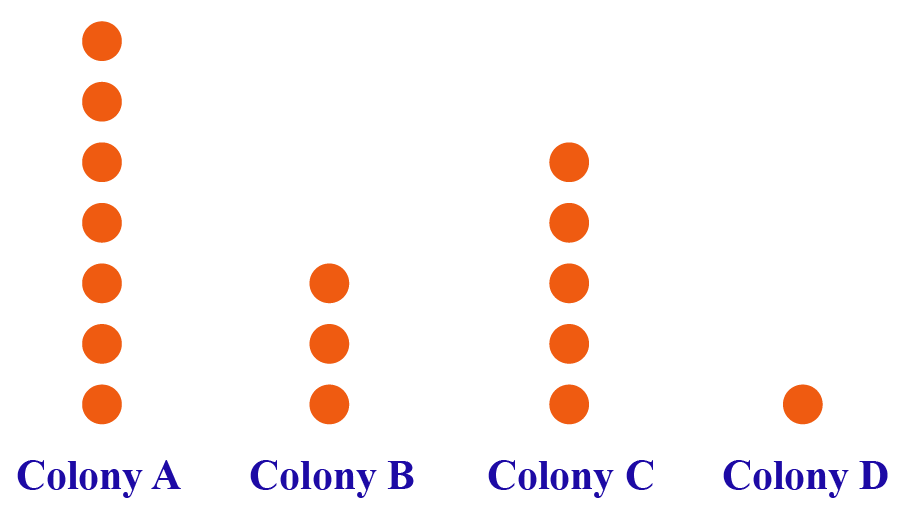Now let's see the number of newborn babies who got a vaccine in each colony.

Colony A has a total of 7 dots, which means that seven babies were vaccinated. Similarly, colony B has three babies, colony C has five babies, and colony D has one baby.

The same dot plot can also be represented as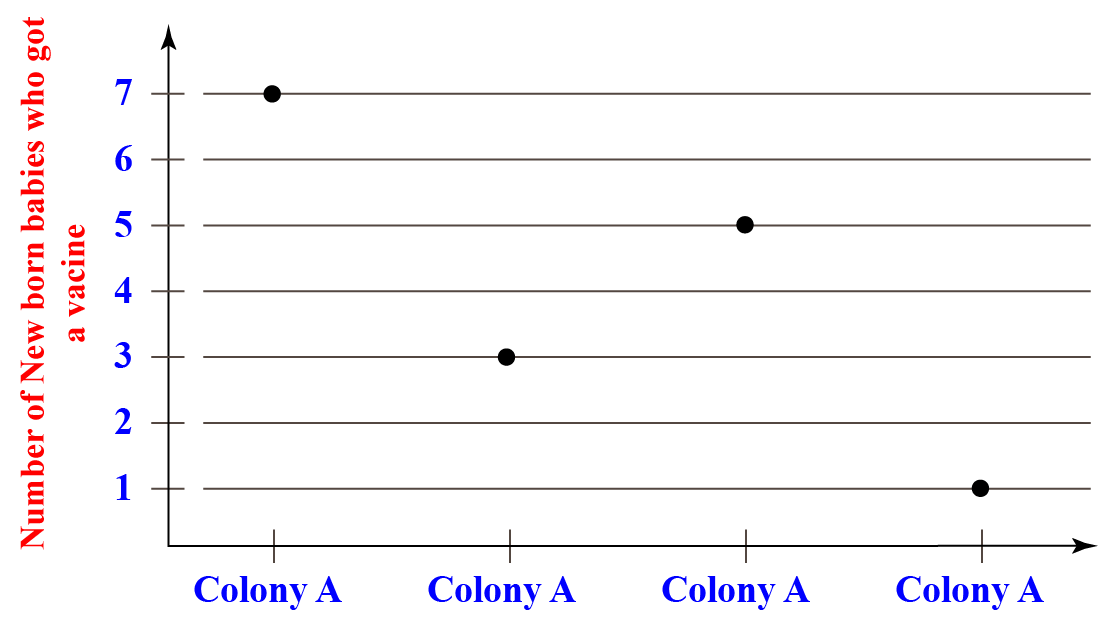Just like how the height of the bars in a bar graph indicates the number of items, the height of the dots is equal to the number of items in that category.

## What Are the Types of Dot Plot?

There are two types of the dot plot

•    Wilkinson dot plot
•    Cleveland dot plot

### Wilkinson Dot Plot

The Wilkinson dot plot represents the distribution of continuous data for example the average temperature in every month of the year.

A histogram is shown in the Wilkinson dot diagram but displays individual data points like points P, Q, R, and S in the below dot plot, these points can take any value like heights of four friends or the number of minutes to finish breakfast by four kids.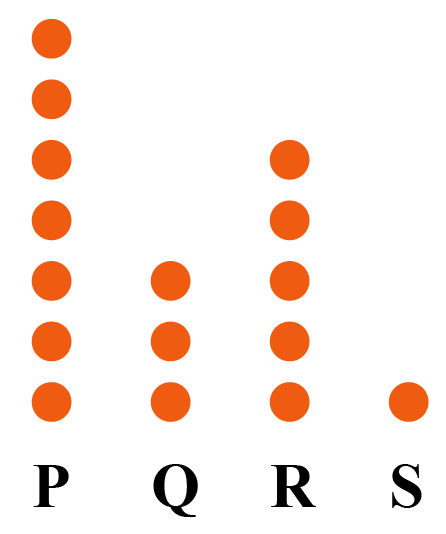### Cleveland Dot Plot

The Cleveland dot plot is a good alternative to a simple bar map if you have more than a few elements.

It doesn't take much to look cluttered on a bar map. Many more values can be used in a dot plot in the same amount of space, and it's also simpler to read.

This type of plot is similar to a bar chart but uses a location instead of the duration of the data encoding values.

Just like how the height of the bar chart represents the number of items, the position of the dot in the graph represents the number of items for that bin or category.

For example, given below is the Cleveland dot plot of literacy rate in post-independent India.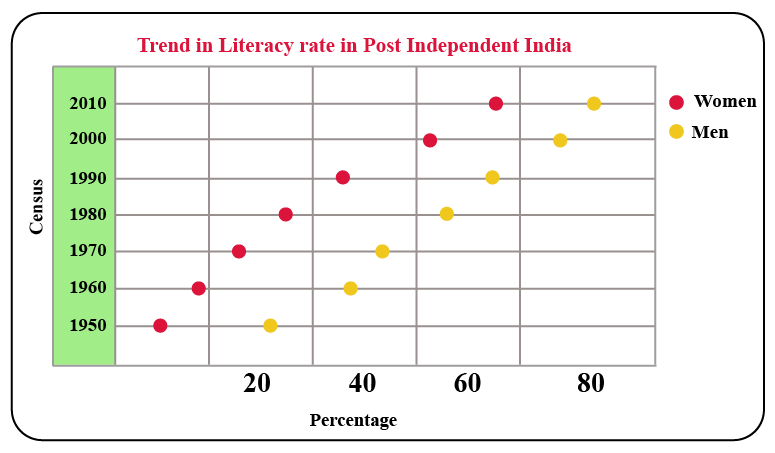## How Do You Construct a Dot Plot?

Let’s take a case to see how to make a dot plot.

There are many ways to draw a dot plot but the best way to draw is by hands.

The data which is given below shows the fuel economy (in miles per gallon) for a sample of 16 cars.

 Vehicle Fuel economy Car 1 16 Car 2 27 Car 3 30 Car 4 31 Car 5 32 Car 6 30 Car 7 31 Car 8 31 Car 9 31 Car 10 24 Car 11 40 Car 12 31 Car 13 31 Car 14 32 Car 15 23 Car 16 29

Now, this given data set definitely includes a wide range of cars It will be exciting to see the plot!

Step 1: Select a scale and set it up.

We are going to create a horizontal scale, and all values must be enclosed. The smallest value is 16 for this data set and the largest is 40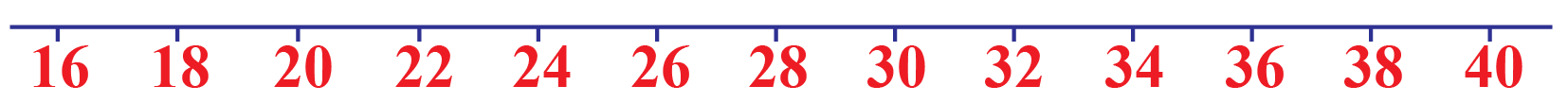You will note that we have chosen to count by 2 rather than 1

This is not important, but it just makes the plot a little more compact. If you like, you can count to 1, 5, or even 10

Step 2: Plot the dots.

Well, this move sounds goofy, but there really isn't any other way to put it.

You will begin to fill in the dots using your scale for this stage.

Keep in mind that "Each value gets a dot & dots are stacked”. To check this, let’s start by plotting only the first row of data.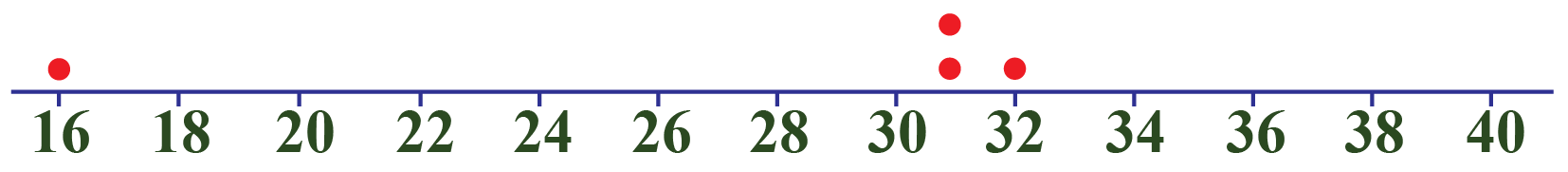You can see that each value is defined by a dot on the plot and that we have two dots set on top of each other. Because there were two cars that got 31 mpg.

Now, with the rest of the details, we can continue the operation.

Keep in mind that while you can't do this perfectly by hand, you should try to make sure that the dots mostly match up.

You do not want broad gaps between dots to make one value seem more prevalent than another.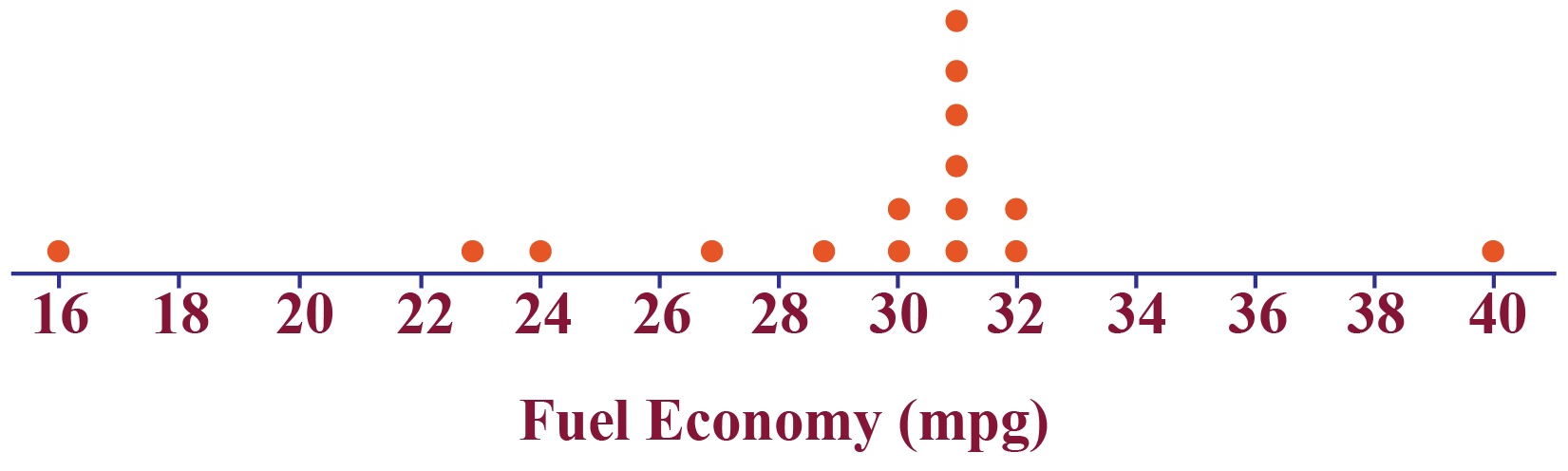That's really it! The dot plot is a great plot to use precisely because it is so easy to create and read for the representation of data.

Note that our purpose is also to convey knowledge to others in statistics. The faster we are able to do this, the better.

Look at the simulation below just fill the values for the corresponding numbers to get a dot plot.

## Where Dot Plot Is Used For?

The dot plot is another type of graphical representation of data like a bar graph, a histogram is also used when data is very small.

So it can be used to convey important information to the viewer or it can be used in schools to display any data.

Dot plots are easy to draw and easy to read so it can be used in most places to display information.

Dot plots are useful for highlighting clusters and gaps.Important Notes
• The dot plot is a graph that displays the distribution of numerical variables where a value is defined by each dot.
• The distribution of continuous data points, such as a histogram, is shown in the Wilkinson dot diagram but displays individual data points rather than bins.
• The Cleveland dot plot shows graphical data elements and displays a continuous variable versus a categorical variable.

## Solved Examples on Dot Plot

 Example 1

The following dot plot shows the height of each toddler at Mrs. Bell's daycare. Each dot represents a different toddler. What is the height of the shortest toddler?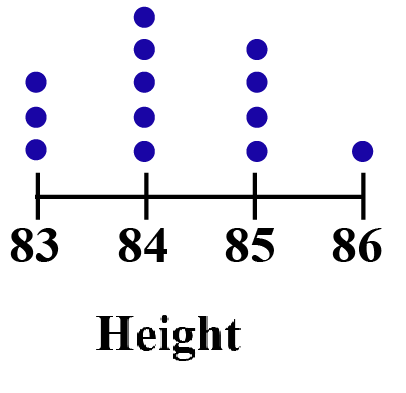Solution

The range on the axis is from 83 - 86
There are three dots at the bin - 83 . which means there is 3 toddler whose height is 83 units.

 $$\therefore$$ the height of shortest toddler is 83units.
 Example 2

The following dot plot illustrates each student's essay score in Mr. Jhonson's class. A different student is represented by each dot. What was the minimum essay score earned by a student? and what is the score earned by the maximum number of students?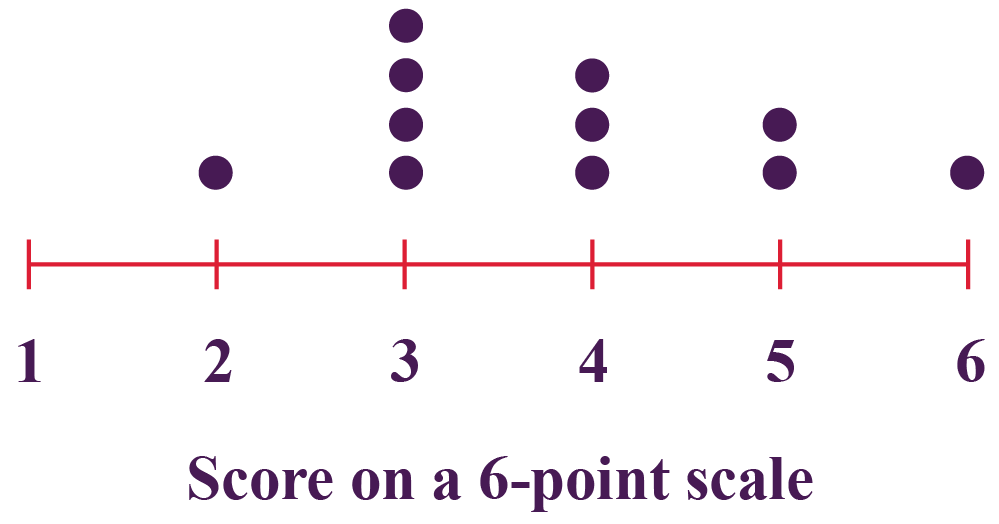Solution

So as per the above data represented in the dot plot.

Shows the data of the number of students who received scores for essays on a 6-point scale.

The minimum essay score that a student received is 2 points.

Four students earned 3 marks, which is the score earned by the maximum number of students.

 $$\therefore$$ minimum essay score that a student received is 3 points and 3 is the marks earned by maximum students.
 Example 3

 Day of the week 1 2 3 4 5 6 7 Number of Hours of Homework 4 5 8 8 5 4 3

The number of hours that students did on homework in one week was recorded in the frequency table above.
Draw a dot plot for the information above.

Solution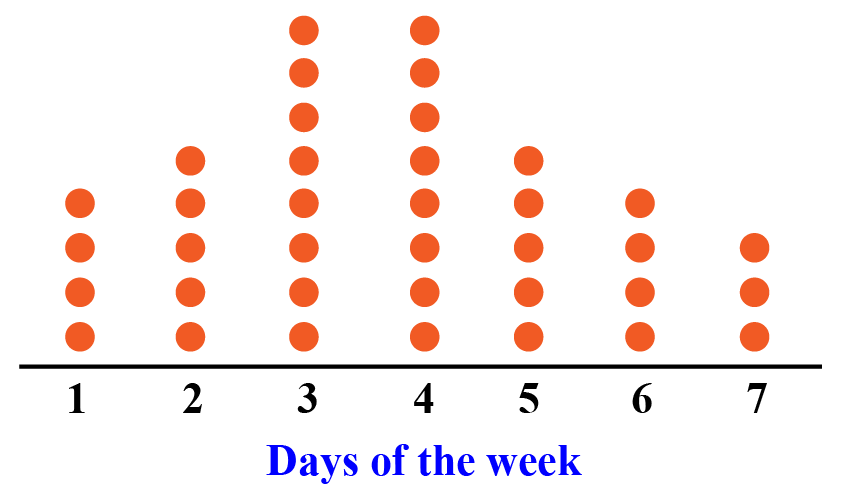Challenging Questions
• As of September 2, 2002, the top 10 home run leaders in the American League have hit 48, 42, 34, 32, 31, 31, 28, 27 and 27 home runs. The top ten home run leaders in the National League had hit 43, 40, 38, 37, 35, 33, 32, 32, and 28 home runs. Make one dot plot from this information. Use it to determine the mean, median, mode, and range. Be sure to explain how you used the dot plot as you determine the measure.
• In Division A of class 9 the height of 10 students are given as 57, 58, 59, 57, 60, 61, 60, 58, 59, & 61(all are in inches). Height of 10 students of Division B of the same grade are 62, 61, 59, 57, 56, 60, 64, 61, 58 & 60(all are in inches). Make one dot plot from this information. Use it to determine the mean, median, mode, and range. Explain how you used the dot plot as you determine the answer.

## Interactive Questions on Dot Plot

Here are a few activities for you to practice.

Select/Type your answer and click the "Check Answer" button to see the result.

## Let's Summarize

This mini-lesson introduced you to the fascinating concept of dot plots. The math journey around the dot plot starts with what a student already knows, and goes on to creatively crafting a fresh concept in the young minds. Done in a way that is not only relatable and easy to grasp, but also will stay with them forever. You have learned about types of dot plots, the use of dot plots, and how to draw a dot plot in this lesson. Here lies the magic with Cuemath.

## About Cuemath

At Cuemath, our team of math experts are dedicated to making learning fun for our favorite readers, the students! Through an interactive and engaging learning-teaching-learning approach, the teachers explore all angles of a topic. Be it simulations, online classes, doubt sessions, or any other form of relation, it’s the logical thinking and smart learning approach that we at Cuemath believe in.

## 1. What is the difference between a line plot and a dot plot?

Line plots use the axis above the numbers that show the amount of each number. Where is dot plot uses dots to show the amount of each number on a number line.

## 2. How do you find the mode on a dot plot?

The bin or container having the maximum number of dots  is the mode of the given set

## 3. Why would you use a dot plot instead of a histogram?

In a dot plot we can illustrate or define each individual data observation but in a histogram data are grouped into classes and then plotted.

More Important Topics
Numbers
Algebra
Geometry
Measurement
Money
Data
Trigonometry
Calculus